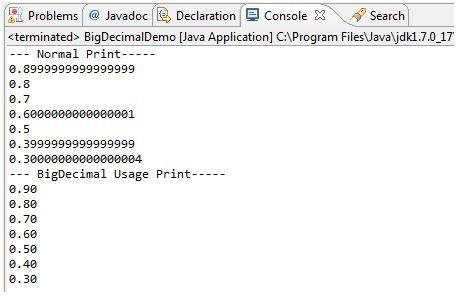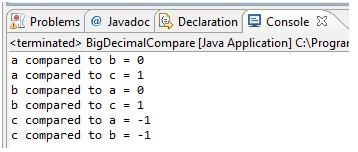Existing user?

Existing user?

## Introduction

Java includes BigDecimal class for performing high-precision arithmetic which can be used in banking or financial domain based application. This class approximately fit into the same category as the “wrapper” classes but has some very useful methods.

This class has methods that provide analogues for the operations that you perform on primitive types. That is, you can do anything with a BigDecimal that you can with an int or float, it’s just that you must use method calls instead of operators. Also, since there’s more involved, the operations will be slower. You’re exchanging speed for accuracy.

BigDecimal is for arbitrary-precision fixed-point numbers; you can use these for accurate monetary calculations. Below Java code explain the concept of accuracy in calculation. One part is doing all calculation using double while other part is having calculation using BigDecimal. Output shows difference between them.

1. import java.math.BigDecimal;
2. public class BigDecimalDemo {
3.     public static void main(String[] argv) {
4.           System.out.println("--- Normal Print-----");
5.           System.out.println(2.00 - 1.1);
6.           System.out.println(2.00 - 1.2);
7.           System.out.println(2.00 - 1.3);
8.           System.out.println(2.00 - 1.4);
9.           System.out.println(2.00 - 1.5);
10.           System.out.println(2.00 - 1.6);
11.           System.out.println(2.00 - 1.7);
12.           System.out.println("--- BigDecimal Usage Print-----");
13.           System.out.println(new BigDecimal("2.00").subtract(new BigDecimal("1.1")));
14.           System.out.println(new BigDecimal("2.00").subtract(new BigDecimal("1.2")));
15.           System.out.println(new BigDecimal("2.00").subtract(new BigDecimal("1.3")));
16.           System.out.println(new BigDecimal("2.00").subtract(new BigDecimal("1.4")));
17.           System.out.println(new BigDecimal("2.00").subtract(new BigDecimal("1.5")));
18.           System.out.println(new BigDecimal("2.00").subtract(new BigDecimal("1.6")));
19.           System.out.println(new BigDecimal("2.00").subtract(new BigDecimal("1.7")));
20.           BigDecimal bd1 = new BigDecimal ("1234.34567");
21.          bd1= bd1.setScale (3, BigDecimal.ROUND_CEILING);
22.           System.out.println(bd1);
23.     }
24. }

## Output## BigDecimal constructors

Construct Type Usage
BigDecimal(BigIntegerval) Takes BigInteger argument to create new BigDecimal value
BigDecimal(BigIntegerunscaledVal, int scale) Translates a BigIntegerunscaled value and an int scale into a BigDecimal.
BigDecimal(BigIntegerunscaledVal, int scale, MathContext mc) Translates a BigIntegerunscaled value and an int scale into a BigDecimal, with rounding according to the context settings.
BigDecimal(BigIntegerval, MathContext mc) Translates a BigInteger into a BigDecimal rounding according to the context settings.
BigDecimal(double d) Takes double type primitive to create new BigDecimal object
BigDecimal(double val, MathContext mc) Translates a double into a BigDecimal, with rounding according to the context settings.
BigDecimal(String s) Takes String object to create new BigDecimal object (can throw number format exception if non-numeric string passed)
BigDecimal(String val, MathContext mc) Translates the string representation of a BigDecimal into a BigDecimal, accepting the same strings as the BigDecimal(String) constructor, with rounding according to the context settings.
BigDecimal(int i) Takes int type primitive to create new BigDecimal object
BigDecimal(int val, MathContext mc) Translates an int into a BigDecimal, with rounding according to the context settings.
BigDecimal(char[] c) Takes char array as argument to generate BigDecimal Object.
BigDecimal(char[] in, int offset, int len) Translates a character array representation of a BigDecimal into a BigDecimal, accepting the same sequence of characters as the BigDecimal(String) constructor, while allowing a sub-array to be specified.
BigDecimal(char[] in, int offset, int len, MathContext mc) Translates a character array representation of a BigDecimal into a BigDecimal, accepting the same sequence of characters as the BigDecimal(String) constructor, while allowing a sub-array to be specified and with rounding according to the context settings.
BigDecimal(char[] in, MathContext mc) Translates a character array representation of a BigDecimal into a BigDecimal, accepting the same sequence of characters as the BigDecimal(String) constructor and with rounding according to the context settings.
BigDecimal(long val) Translates a long into a BigDecimal.
BigDecimal(long val, MathContext mc) Translates a long into a BigDecimal, with rounding according to the context settings.

## BigDecimal important methods

Method Name Description
abs() Returns a BigDecimal whose value is the absolute value of this BigDecimal.
abs(MathContext mc) Returns a BigDecimal whose value is the absolute value of this BigDecimal, with rounding according to the context settings.
add(BigDecimal augend) Returns a BigDecimal whose value is (this + augend), and whose scale is max(this.scale(), augend.scale()).
add(BigDecimal augend, MathContext mc) Returns a BigDecimal whose value is (this + augend), with rounding according to the context settings.
byteValueExact() Converts this BigDecimal to a byte, checking for lost information.
compareTo(BigDecimal val) Compares this BigDecimal with the specified BigDecimal.
divide(BigDecimal divisor) Returns a BigDecimal whose value is (this / divisor) if the exact quotient cannot be represented (because it has a non-terminating decimal expansion) an ArithmeticException is thrown.
divide(BigDecimal divisor, int roundingMode) Returns a BigDecimal whose value is (this / divisor), and whose scale is this.scale().
divide(BigDecimal divisor, int scale, int roundingMode) Returns a BigDecimal whose value is (this / divisor), and whose scale is as specified.
divide(BigDecimal divisor, int scale, RoundingModeroundingMode) Returns a BigDecimal whose value is (this / divisor), and whose scale is as specified.
divide(BigDecimal divisor, MathContext mc) Returns a BigDecimal whose value is (this / divisor), with rounding according to the context settings.
divide(BigDecimal divisor, RoundingModeroundingMode) Returns a BigDecimal whose value is (this / divisor), and whose scale is this.scale().
divideAndRemainder(BigDecimal divisor) Returns a two-element BigDecimal array containing the result of divideToIntegralValue followed by the result of remainder on the two operands.
divideAndRemainder(BigDecimal divisor, MathContext mc) Returns a two-element BigDecimal array containing the result of divideToIntegralValue followed by the result of remainder on the two operands calculated with rounding according to the context settings.
divideToIntegralValue(BigDecimal divisor) Returns a BigDecimal whose value is the integer part of the quotient (this / divisor) rounded down.
divideToIntegralValue(BigDecimal divisor, MathContext mc) Returns a BigDecimal whose value is the integer part of (this / divisor).
doubleValue() Converts this BigDecimal to a double.
equals(Object x) Compares this BigDecimal with the specified Object for equality.
floatValue() Converts this BigDecimal to a float.
hashCode() Returns the hash code for this BigDecimal.
intValue() Converts this BigDecimal to an int.
intValueExact() Converts this BigDecimal to an int, checking for lost information.
longValue() Converts this BigDecimal to a long.
longValueExact() Converts this BigDecimal to a long, checking for lost information.
max(BigDecimal val) Returns the maximum of this BigDecimal and val.
min(BigDecimal val) Returns the minimum of this BigDecimal and val.
movePointLeft(int n) Returns a BigDecimal which is equivalent to this one with the decimal point moved n places to the left.
movePointRight(int n) Returns a BigDecimal which is equivalent to this one with the decimal point moved n places to the right.
multiply(BigDecimal multip) Returns a BigDecimal whose value is (this × multip), and whose scale is (this.scale() + multiplicand.scale()).
multiply(BigDecimal multiplicand, MathContext mc) Returns a BigDecimal whose value is (this × multiplicand), with rounding according to the context settings.
negate() Returns a BigDecimal whose value is (-this), and whose scale is this.scale().
negate(MathContext mc) Returns a BigDecimal whose value is (-this), with rounding according to the context settings.
plus() Returns a BigDecimal whose value is (+this), and whose scale is this.scale().
plus(MathContext mc) Returns a BigDecimal whose value is (+this), with rounding according to the context settings.
pow(int n) Returns a BigDecimal whose value is (thisn), The power is computed exactly, to unlimited precision.
pow(int n, MathContext mc) Returns a BigDecimal whose value is (thisn).
precision() Returns the precision of this BigDecimal.
remainder(BigDecimal divisor) Returns a BigDecimal whose value is (this % divisor).
remainder(BigDecimal divisor, MathContext mc) Returns a BigDecimal whose value is (this % divisor), with rounding according to the context settings.
round(MathContext mc) Returns a BigDecimal rounded according to the MathContext settings.
scale() Returns the scale of this BigDecimal.
scaleByPowerOfTen(int n) Returns a BigDecimal whose numerical value is equal to (this * 10n).
setScale(int newScale) Returns a BigDecimal whose scale is the specified value, and whose value is numerically equal to this BigDecimal's.
setScale(int newScale, int roundingMode) Returns a BigDecimal whose scale is the specified value,and whose unscaled value is determined by multiplying or dividing this BigDecimal'sunscaled value by the appropriate power of ten to maintain its overall value.
setScale(int newScale, RoundingModeroundingMode) Returns a BigDecimal whose scale is the specified value, and whose unscaled value is determined by multiplying or dividing this BigDecimal'sunscaled value by the appropriate power of ten to maintain its overall value.
shortValueExact() Converts this BigDecimal to a short, checking for lost information.
signum() Returns the signum function of this BigDecimal.
stripTrailingZeros() Returns a BigDecimal which is numerically equal to this one but with any trailing zeros removed from the representation.
subtract(BigDecimal subtrahend) Returns a BigDecimal whose value is (this - subtrahend), and whose scale is max(this.scale(), subtrahend.scale()).
subtract(BigDecimal subtrahend, MathContext mc) Returns a BigDecimal whose value is (this - subtrahend), with rounding according to the context settings.
toBigInteger() Converts this BigDecimal to a BigInteger.
toBigIntegerExact() Converts this BigDecimal to a BigInteger, checking for lost information.
toEngineeringString() Returns a string representation of this BigDecimal, using engineering notation if an exponent is needed.
toPlainString() Returns a string representation of this BigDecimal without an exponent field.
ulp() Returns the size of an ulp, a unit in the last place, of this BigDecimal.
unscaledValue() Returns a BigInteger whose value is the unscaled value of this BigDecimal.

### Limiting a number to a certain degree of precision

Let us say, we would like to calculate something, up to 3 decimal places, no more and no less, and independent of the number we used to instantiatethe BigDecimal. In that case, we will have to specify two properties of a BigDecimal object. The rounding mode and the scale. The scale definesthe number of decimal places we want, and the rounding mode specifies how we would like to round a floating point number. As an example, we willmodify the task above as follows :

"After you have created the program, your bosses at NASA have changed their minds, and they now want the result accurate up to no more than 3 decimalplaces. You are now required to change your program."

```BigDecimal bd1 = new BigDecimal ("1234.34567");
bd1= bd1.setScale (3, BigDecimal.ROUND_CEILING);
System.out.println(bd1);
```

Output would be 1234.346

### Comparing BigDecimals

As with primitive numeric data types, one will sometimes need to compare BigDecimals with each other. To do so, one will have to usethe "x.compareTo( BigDecimal bd)" method. (Note: Do not use the .equals() method. It is a common mistake to do so, as it comparestwo pointers to determine of they point to the same object, and has nothing to do with numeric equality).

The compareTo method works asfollows:lets say we make the call "x.compareTo(y)". The result of this call will be : (as in the comparable interface)

```-1 : if x < y
0 : if x == y
+1: if x > y
```

1. import java.math.BigDecimal;
2. public class BigDecimalCompare {
3.      public static void main(String[]args){
4.          BigDecimal a = new BigDecimal("31234");
5.          BigDecimal b = new BigDecimal("31234.00");
6.          BigDecimal c = new BigDecimal("-21234");
7.          System.out.println("a compared to b = "+a.compareTo(b));
8.          System.out.println("a compared to c = "+a.compareTo(c));
9.          System.out.println("b compared to a = "+b.compareTo(a));
10.          System.out.println("b compared to c = "+b.compareTo(c));
11.          System.out.println("c compared to a = "+c.compareTo(a));
12.          System.out.println("c compared to b = "+c.compareTo(b));
13.
14.     }
15. }

## Output## Summary

• BigDecimal class should be used when we need accuracy and particular scale in our results.
• BigDecimal is similar to other wrapper classes having specific methods for addition, subtraction, multiplication and division.
• This wrapper class operations are slightly slower compare to primitive types.

Garbage Collection in Java
Java ArrayList Class

<< Previous